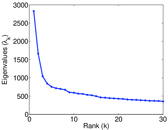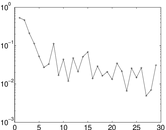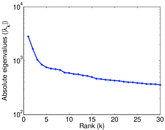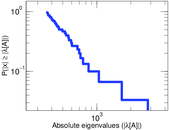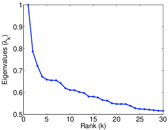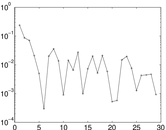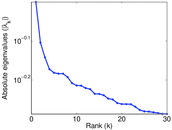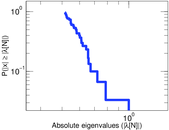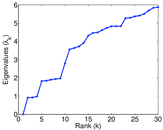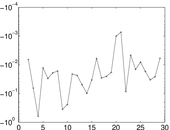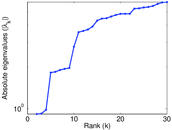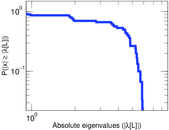# Enron words

This is the bipartite document–word dataset of Enron words. Left nodes are documents and right nodes are words. Edge weights are multiplicities.

 Code `EN` Internal name `bag-enron` Name Enron words Data source http://archive.ics.uci.edu/ml/datasets/Bag+of+Words AvailabilityDataset is available for download Consistency checkDataset passed all tests Category Text network Node meaning Document, word Edge meaning Occurrence Network formatBipartite, undirected Edge typeUnweighted, multiple edges

## Statistics

 Size n = 67,960 Left size n1 = 39,861 Right size n2 = 28,099 Volume m = 6,412,172 Unique edge count m̿ = 3,710,420 Wedge count s = 3,214,624,476 Claw count z = 2,510,007,422,598 Cross count x = 2,191,825,474,071,012 4-Tour count T4 = 376,634,510,028 Maximum degree dmax = 7,190 Maximum left degree d1max = 2,120 Maximum right degree d2max = 7,190 Average degree d = 188.704 Average left degree d1 = 160.863 Average right degree d2 = 228.199 Fill p = 0.003 312 71 Average edge multiplicity m̃ = 1.728 15 Size of LCC N = 67,960 Diameter δ = 6 50-Percentile effective diameter δ0.5 = 2.492 21 90-Percentile effective diameter δ0.9 = 3.606 21 Median distance δM = 3 Mean distance δm = 2.992 72 Gini coefficient G = 0.707 894 Balanced inequality ratio P = 0.224 254 Left balanced inequality ratio P1 = 0.225 645 Right balanced inequality ratio P2 = 0.156 346 Relative edge distribution entropy Her = 0.897 344 Power law exponent γ = 1.269 14 Tail power law exponent γt = 1.991 00 Degree assortativity ρ = −0.174 109 Degree assortativity p-value pρ = 0.000 00 Spectral separation |λ1[A] / λ2[A]| = 1.700 69 Controllability C = 14,724 Relative controllability Cr = 0.216 657

## Plots

### Fruchterman–Reingold graph drawing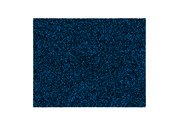### Degree distribution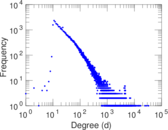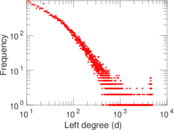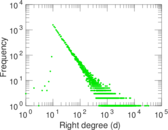### Cumulative degree distribution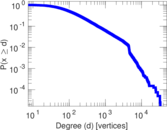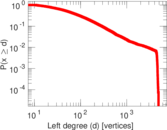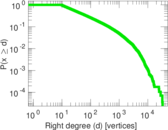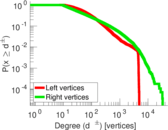### Lorenz curve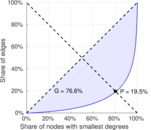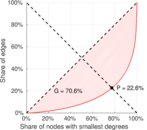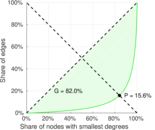### Spectral distribution of the adjacency matrix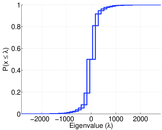### Spectral distribution of the normalized adjacency matrix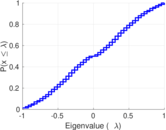### Spectral distribution of the Laplacian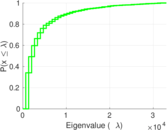### Spectral graph drawing based on the adjacency matrix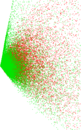### Spectral graph drawing based on the Laplacian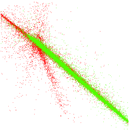### Spectral graph drawing based on the normalized adjacency matrix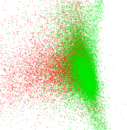### Degree assortativity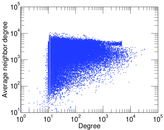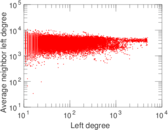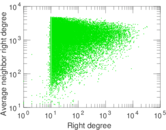### Zipf plot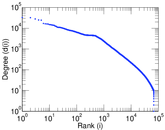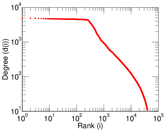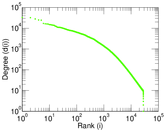### Hop distribution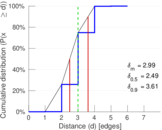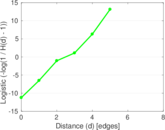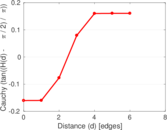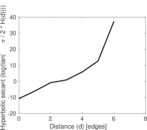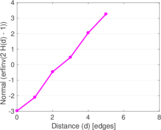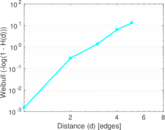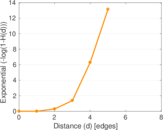### Edge weight/multiplicity distribution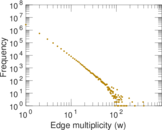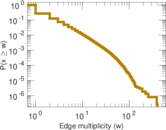### Matrix decompositions plots